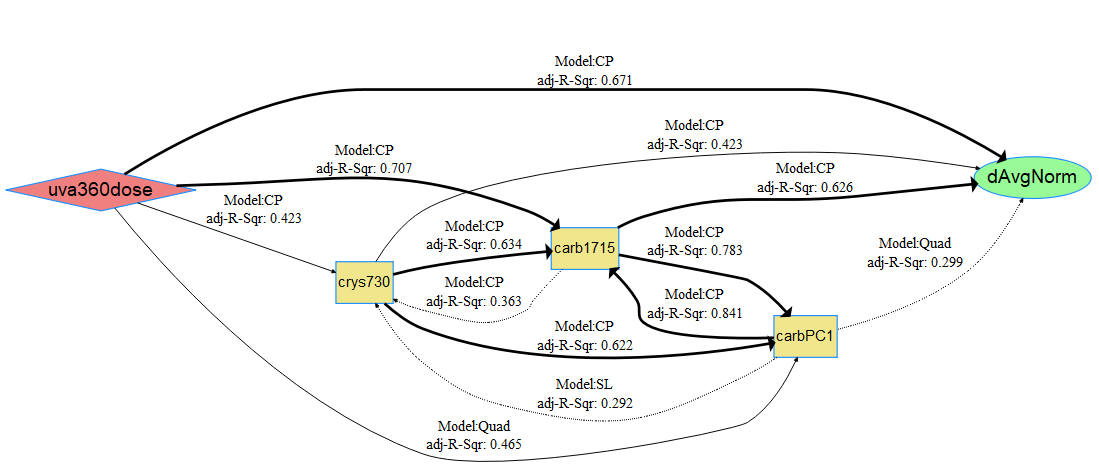# Quantifying and Modeling Photovoltaic Backsheet Cracking

## Data Description

A dataset of cracks in photovoltaic backsheets with inner layers of either ethylene-vinyl acetate or polyethylene exposed to 4,000 hours of continuous UVA irradiance with an intensity of 1.55 $$w/m^2$$ at 340 $$nm$$ and a chamber temperature of 70$$^\circ$$C. See the associated journal article in Polymer Degradation and Stability for details on obtaining quantitative crack measurements (Klinke et al. 2018).

130 observations of 5 variables are included:

• Response - dAvgNorm: average crack depth normalized by the backsheet’s inner layer thickness, dimensionless.
• Stress - uva360dose: cumulative, integrated irradiance dose received by the sample for all wavelengths $$<$$ 360 $$nm$$, in $$MJ/m^2$$.
• Mechanism - crys730: percent crystallinity of polyethylene regions determined from the ratio of Fourier transform infrared (FTIR) spectroscopy peak intensities at 731 and 720 $$cm^{-1}$$ (Huang, Hong, and Urban 1992), dimensionless.
• Mechanism - carb1715: ketone carbonyl index determined from the ratio of FTIR peak intensities at 1715 and 2851 $$cm^{-1}$$, dimensionless.
• Mechanism - carbPC1: 1st principal component score for the carbonyl region between 1500 and 1800 $$cm^{-1}$$.

## Load data and run code to build netSEM

## Load the crack data set and preview column metadata
data(crack)
?crack

## Run netSEM
ans <- netSEMm(crack)

## Subset dataset with three cutoffs
res <- subsetData(ans, cutoff=c(0.4, 0.5, 0.6))

## Plot the network model
plot(ans, res)

## Network diagram for data

dAvgNorm is the endogenous and all other variables are considered as exagenous. Based on the plot, the strongest pathway appears to be uva360dose $$\rightarrow$$ carb1715 $$\rightarrow$$ dAvgNorm. This can be confirmed using the pathwayRMSE() function.